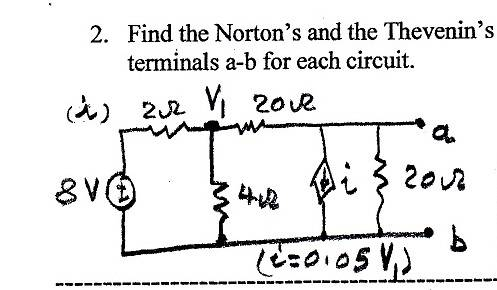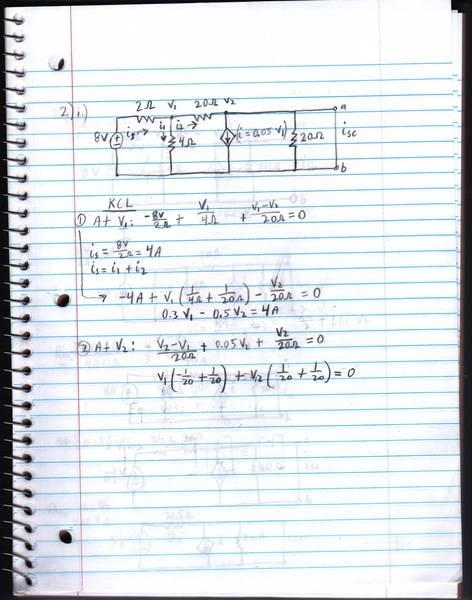# Help with KCL/Thevenin Problem - Urgently Needed

I tried solving this problem using KCL, but V1 keeps adding up to zero for my second set of equations. I'm not sure what I'm doing wrong. Any help is much appreciated.

1. Homework Statement

I need to find the Thevenin equivalent circuit. I chose to find the Isc and the Voc by using KCL, since I don't see another way.KCL

## The Attempt at a Solution

[/B]
You can see for equation 2, my V1 equals zero due to the dependent current source relation. Not sure how to get around this.NascentOxygen
Staff Emeritus
Right at the start you write is = 8v/2Ω

Why do you say this?

Right at the start you write is = 8v/2Ω

Why do you say this?

My mistake. That should read (v1-8)/2.

I'm still stuck on the second equation however since v1 equals zero. Am I missing something obvious here?

CWatters
Homework Helper
Gold Member
My mistake. That should read (v1-8)/2.

I don't think that's right either.

Edit: Do you mean "Is = (v1-8)/2" or that term in equation should be (v1-8)/2 ? The latter is correct.

Last edited:
I don't think that's right either.

Edit: Do you mean "Is = (v1-8)/2" or that term in equation should be (v1-8)/2

That's for the term in the first KCL equation. But V1 in the second equation equals zero which has me stuck. I can't set up my equations to find any of the unknowns.

CWatters
Homework Helper
Gold Member
I think you mean V2 = 0 not V1.

I think you mean V2 = 0 not V1.

I mean (-1/20 + 1/20) zeroes out V1 at node 2, since the dependent source is equal to 0.05V1

NascentOxygen
Staff Emeritus
You can see for equation 2, my V1 equals zero due to the dependent current source relation. Not sure how to get around this.
I see the problem. They cunningly have the current source always draining the entire current sourced through the 20Ω.

An alternative method to determine the impedance at ab is to apply a test voltage across ab and calculate how much current is drawn from that source. I guess that means the Thévenin equivalent will then be a simple resistance.

I see the problem. They cunningly have the current source always draining the entire current sourced through the 20Ω.

An alternative method to determine the impedance at ab is to apply a test voltage across ab and calculate how much current is drawn from that source. I guess that means the Thévenin equivalent will then be a simple resistance.

I'm not quite sure what you mean :/

CWatters